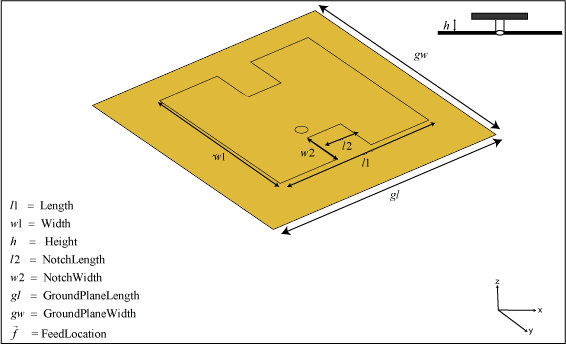# patchMicrostripHnotch

H-shaped microstrip patch antenna

## Description

Use the `patchMicrostripHnotch` object to create an H-shaped microstrip patch antenna. The default patch is centered at the origin with the feedpoint along the length. By default, the dimensions are chosen for an operating frequency of 3.49 GHz for air or 2.61 GHz for Teflon.## Creation

### Syntax

``ant = patchMicrostripHnotch``
``ant = patchMicrostripHnotch(Name,Value)``

### Description

example

````ant = patchMicrostripHnotch` creates an H-shaped microstrip patch antenna. ```

example

````ant = patchMicrostripHnotch(Name,Value)` sets properties using one or more name-value pairs. For example, `ant = patchMicrostripHnotch('Width',0.2)` creates a microstrip H-patch with a patch width of 0.2 m. Enclose each property name in quotes.```

## Properties

expand all

Patch length along the X-axis, specified as a scalar in meters.

Example: `'Length',0.0450`

Example: `ant.Length = 0.0450`

Data Types: `double`

Patch width along the Y-axis, specified as a scalar in meters.

Example: `'Width',0.0500`

Example: `ant.Width = 0.0500`

Data Types: `double`

Notch length along the X-axis, specified as a scalar in meters.

Example: `'NotchLength',0.0200`

Example: `ant.NotchLength = 0.0200`

Data Types: `double`

Notch width along the Y-axis, specified as a scalar in meters.

Example: `'NotchWidth',0.00600`

Example: `ant.NotchWidth = 0.00600`

Data Types: `double`

Patch height above the ground plane along the Z-axis, specified as a scalar in meters.

Example: `'Height',0.00500`

Example: `ant.Height = 0.00500`

Data Types: `double`

Type of dielectric material used as a substrate, specified as a dielectric object. For more information see, `dielectric`.

Example: `d = dielectric('FR4');` `ant = patchMicrostripHnotch('Substrate',d)`

Example: `d = dielectric('FR4');` `ant = patchMicrostripHnotch;` `ant.Substrate = d;`

Data Types: `string` | `char`

Ground plane length along the X-axis, specified as a scalar in meters. Setting the ground plane length to `Inf` uses the infinite ground plane technique for antenna analysis.

Example: `'GroundPlaneLength',120e-3`

Example: `ant.GroundPlaneLength = 120e-3`

Data Types: `double`

Ground plane width along the Y-axis, specified as a scalar in meters. Setting the ground plane width to `Inf` uses the infinite ground plane technique for antenna analysis.

Example: `'GroundPlaneWidth',120e-3`

Example: `ant.GroundPlaneWidth = 120e-3`

Data Types: `double`

Signed distance of the patch from the origin, specified as a two-element real-valued vector with each element unit in meters. Use this property to adjust the location of the patch relative to the ground plane. Distances are measured along the length and width of the ground plane.

Example: `'PatchCenterOffset',[0.01 0.01]`

Example: ```ant.PatchCenterOffset = [0.01 0.01]```

Data Types: `double`

Signed distance of the feed from the origin, specified as a two-element real-valued vector with each element unit in meters. Use this property to adjust the location of the feedpoint relative to the ground plane and patch. Distances are measured along the length and width of the ground plane.

Example: `'FeedOffset',[0.01 0.01]`

Example: `ant.FeedOffset = [0.01 0.01]`

Data Types: `double`

Feed diameter, specified as a scalar in meters.

Example: `'FeedDiameter',0.0600`

Example: `ant.FeedDiameter = 0.0600`

Data Types: `double`

Type of the metal used as a conductor, specified as a metal material object. You can choose any metal from the `MetalCatalog` or specify a metal of your choice. For more information, see `metal`. For more information on metal conductor meshing, see Meshing.

Example: ```m = metal('Copper'); 'Conductor',m```

Example: ```m = metal('Copper'); ant.Conductor = m```

Lumped elements added to the antenna feed, specified as a lumped element object. You can add a load anywhere on the surface of the antenna. By default, the load is at the origin. For more information, see `lumpedElement`.

Example: `'Load',lumpedelement`, where `lumpedelement` is the object handle for the load created using `lumpedElement`.

Example: ```ant.Load = lumpedElement('Impedance',75)```

Tilt angle of the antenna, specified as a scalar or vector with each element unit in degrees. For more information, see Rotate Antennas and Arrays.

Example: `'Tilt',90`

Example: `ant.Tilt = 90`

Example: `'Tilt',[90 90]`,`'TiltAxis',[0 1 0;0 1 1]` tilts the antenna at 90 degrees about the two axes defined by the vectors.

Note

The `wireStack` antenna object only accepts the dot method to change its properties.

Data Types: `double`

Tilt axis of the antenna, specified as:

• Three-element vector of Cartesian coordinates in meters. In this case, each coordinate in the vector starts at the origin and lies along the specified points on the X-, Y-, and Z-axes.

• Two points in space, each specified as three-element vectors of Cartesian coordinates. In this case, the antenna rotates around the line joining the two points in space.

• A string input describing simple rotations around one of the principal axes, 'X', 'Y', or 'Z'.

Example: `'TiltAxis',[0 1 0]`

Example: `'TiltAxis',[0 0 0;0 1 0]`

Example: `ant.TiltAxis = 'Z'`

Note

The `wireStack` antenna object only accepts the dot method to change its properties.

Data Types: `double`

## Object Functions

 `show` Display antenna or array structure; display shape as filled patch `axialRatio` Axial ratio of antenna `beamwidth` Beamwidth of antenna `charge` Charge distribution on metal or dielectric antenna or array surface `current` Current distribution on metal or dielectric antenna or array surface `design` Design prototype antenna or arrays for resonance at specified frequency `efficiency` Radiation efficiency of antenna `EHfields` Electric and magnetic fields of antennas; Embedded electric and magnetic fields of antenna element in arrays `impedance` Input impedance of antenna; scan impedance of array `mesh` Mesh properties of metal or dielectric antenna or array structure `meshconfig` Change mesh mode of antenna structure `optimize` Optimize antenna or array using SADEA optimizer `pattern` Radiation pattern and phase of antenna or array; Embedded pattern of antenna element in array `patternAzimuth` Azimuth pattern of antenna or array `patternElevation` Elevation pattern of antenna or array `returnLoss` Return loss of antenna; scan return loss of array `sparameters` S-parameter object `vswr` Voltage standing wave ratio of antenna

## Examples

collapse all

Create and view a microstrip patch H-notch with default property values.

```ant = patchMicrostripHnotch; show(ant)```Create an H-shaped patch with dielectric substrate of permittivity 2.33.

```ant = patchMicrostripHnotch('Substrate',dielectric('EpsilonR',2.33,'LossTangent',0.0012)); show(ant);```